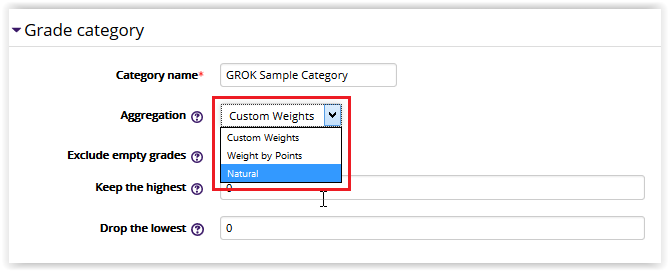### Moodle 3: Gradebook: Editing Aggregation Methods

#### Aggregation Methods

There are 3 category Aggregation Methods to choose from for determining how Moodle calculates a category grade total.  For more information on the Moodle Gradebook, see the Moodle 3: Gradebook: Overview article.

##### 1. Custom Weights

This method allows you to set custom numeric weights that determine the relative value of a grade item (as compared to the value of other items in the category.) By default all items have a weight of 1 and are averaged equally together.  To change the weight, erase the existing "1.0," and input the desired number of weight for each graded item or category in consistent format.  For example, 0.2 and 20 would both represent 20% if all numbers are added up as 1 or 100. This method "normalizes" all grade items, putting them on a 100 point scale. For example, a score of 9 on a 10-point quiz would be the equivalent of a score of 90 on a 100-point exam. Grade items that do not yet contain a value (blank grades) are excluded from the calculation of a category total.

##### 2. Weight by Points

This method determines the relative weight of grade items based on the Maximum Grade of each item. For example, a 50-point test would be worth half as much as a 100-point exam. Grade items that do not yet contain a value (blank grades) are excluded from the calculation of a category total.

##### 3. Natural

This method is the sum of all grade values, scaled by weight. Scale grades are ignored. This is the only type that does not convert the grades to percentages internally. The Maximum grade of associated category item is calculated automatically as a sum of maximums from all aggregated items.

#### How to Change the Aggregation Method?

1. Login to Moodle and select the desired course.

2. In the Administration block on the left side of the screen, click Gradebook Setup.3. Once Gradebook Setup opens, select Edit Settings in the Actions column.

NOTE: You must do this for the overall Gradebook Category, NOT the graded item.4. Locate the Aggregation fieldbox under the Grade Category dropdown. Choose your method from the list provided.5. Click Save Changes at the bottom of the screen.18499
5/21/2020 6:46:44 PM